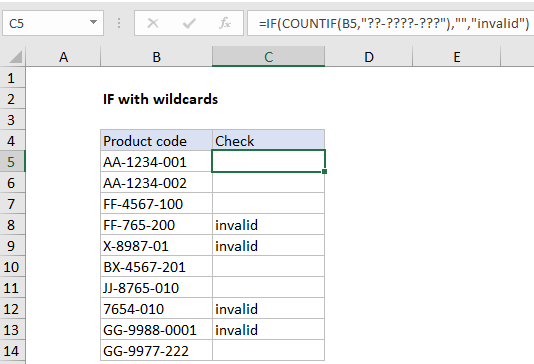## Excel Office

Excel How Tos, Tutorials, Tips & Tricks, Shortcuts

# IF with wildcards in Excel

This tutorial shows how to calculate IF with wildcards in Excel using the example below;

## Formula

`=IF(COUNTIF(A1,"??-????-???"),"","invalid")`## Explanation

The IF function doesn’t support wildcards, but you can combine IF with COUNTIF or COUNTIF to get basic wildcard functionality. In the example shown, the formula in C5 is:

`=IF(COUNTIF(B5,"??-????-???"),"","invalid")`

### How this formula works

Unlike several other frequently used functions, the IF function does not support wildcards. However, you can use the COUNTIF or COUNTIFS functions inside the logical test of IF for basic wildcard functionality.

Also See:   How to test a range for numbers in Excel

In the example shown, the formula in C5 is:

`=IF(COUNTIF(B5,"??-????-???"),"","invalid")`

Working from the inside out, the logical test inside the IF function is based on the COUNTIF function:

`COUNTIF(B5,"??-????-???")`

Here, COUNTIF counts cells that match the pattern “??-????-???”, but since the range is just one cell, the answer is always 1 or zero. The question mark wildcard (?) means “one character”, so COUNTIF returns the number 1 when the text consists of 11 characters with two hyphens, as described by the pattern. If cell contents do not match this pattern, COUNTIF returns zero.

Also See:   Countif function in Excel

When the count is 1, the IF function returns an empty string (“”). When the count is zero, IF returns the text “invalid”. This works because of boolean logic, where the number 1 is evaluated as TRUE and the number zero is evaluated as FALSE.

### Alternative with SEARCH function

Another way to use wildcards with the IF function is to combine the SEARCH and ISNUMBER functions to create a logical test. This works because the SEARCH function supports wildcards. However, SEARCH and ISNUMBER together automatically perform a “contains-type” match, so wildcards aren’t always needed.

Also See:   Count long numbers without COUNTIF in Excel